# Propositional and FirstOrder Logic Chapter 7 47 8

• Slides: 36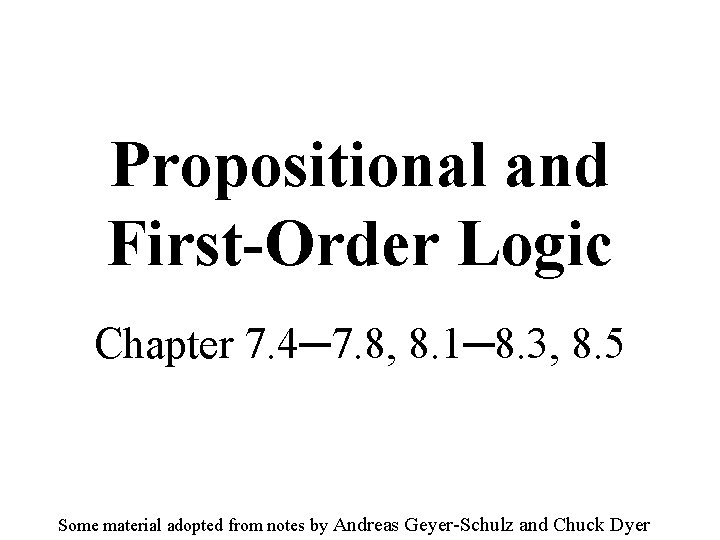Propositional and First-Order Logic Chapter 7. 4─7. 8, 8. 1─8. 3, 8. 5 Some material adopted from notes by Andreas Geyer-Schulz and Chuck Dyer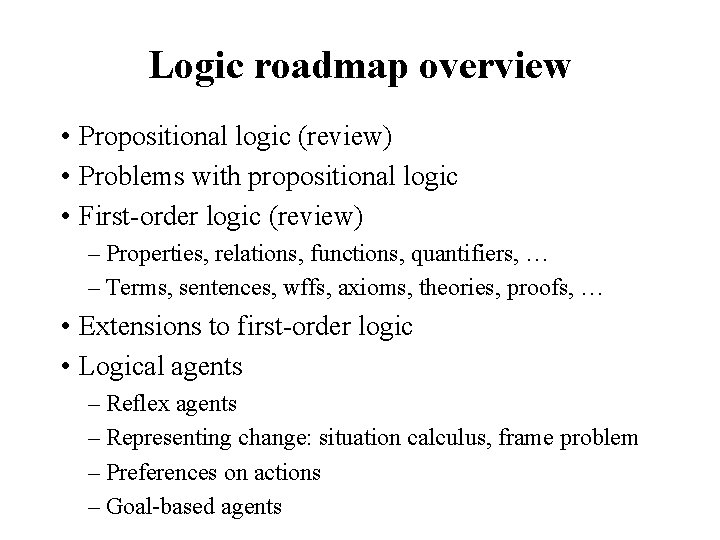Logic roadmap overview • Propositional logic (review) • Problems with propositional logic • First-order logic (review) – Properties, relations, functions, quantifiers, … – Terms, sentences, wffs, axioms, theories, proofs, … • Extensions to first-order logic • Logical agents – Reflex agents – Representing change: situation calculus, frame problem – Preferences on actions – Goal-based agentsDisclaimer “Logic, like whiskey, loses its beneficial effect when taken in too large quantities. ” - Lord Dunsany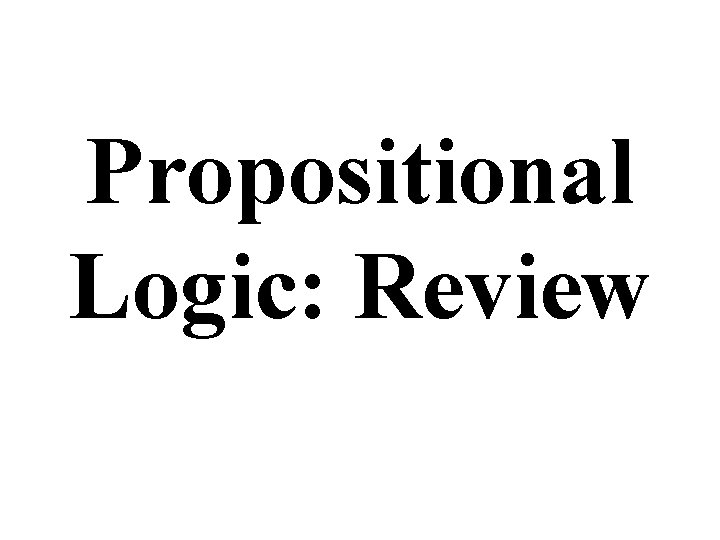Propositional Logic: Review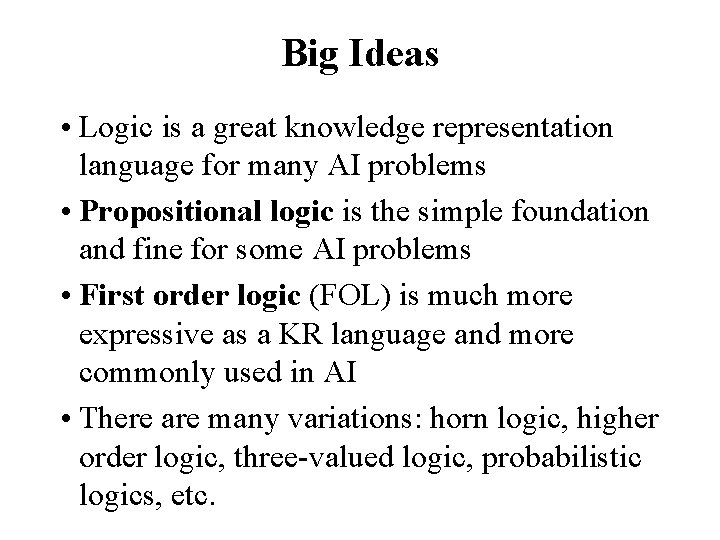Big Ideas • Logic is a great knowledge representation language for many AI problems • Propositional logic is the simple foundation and fine for some AI problems • First order logic (FOL) is much more expressive as a KR language and more commonly used in AI • There are many variations: horn logic, higher order logic, three-valued logic, probabilistic logics, etc.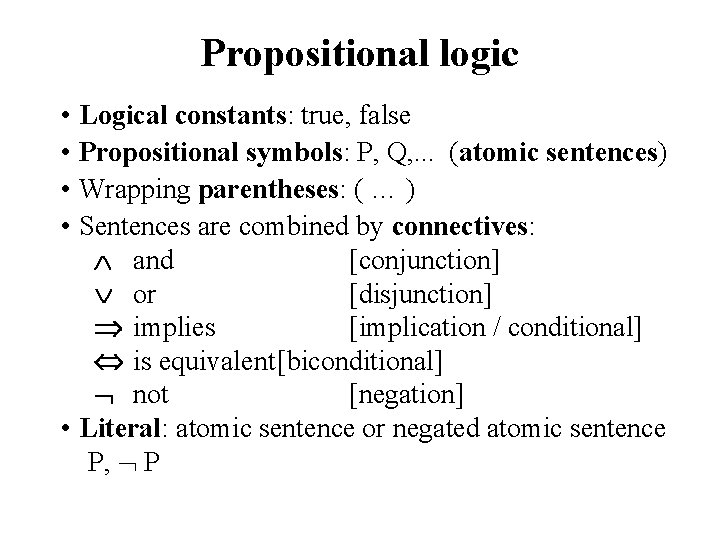Propositional logic • Logical constants: true, false • Propositional symbols: P, Q, . . . (atomic sentences) • Wrapping parentheses: ( … ) • Sentences are combined by connectives: and [conjunction] or [disjunction] implies [implication / conditional] is equivalent[biconditional] not [negation] • Literal: atomic sentence or negated atomic sentence P, P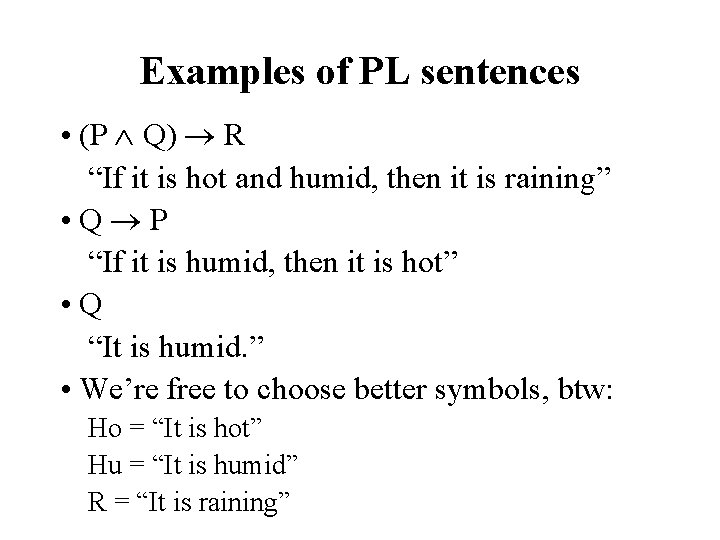Examples of PL sentences • (P Q) R “If it is hot and humid, then it is raining” • Q P “If it is humid, then it is hot” • Q “It is humid. ” • We’re free to choose better symbols, btw: Ho = “It is hot” Hu = “It is humid” R = “It is raining”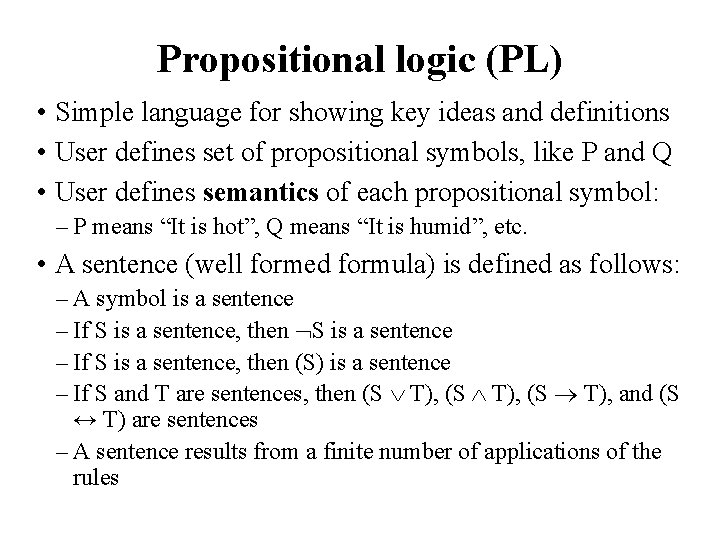Propositional logic (PL) • Simple language for showing key ideas and definitions • User defines set of propositional symbols, like P and Q • User defines semantics of each propositional symbol: – P means “It is hot”, Q means “It is humid”, etc. • A sentence (well formed formula) is defined as follows: – A symbol is a sentence – If S is a sentence, then S is a sentence – If S is a sentence, then (S) is a sentence – If S and T are sentences, then (S T), and (S ↔ T) are sentences – A sentence results from a finite number of applications of the rulesSome terms • The meaning or semantics of a sentence determines its interpretation • Given the truth values of all symbols in a sentence, it can be “evaluated” to determine its truth value (True or False) • A model for a KB is a possible world – an assignment of truth values to propositional symbols that makes each sentence in the KB True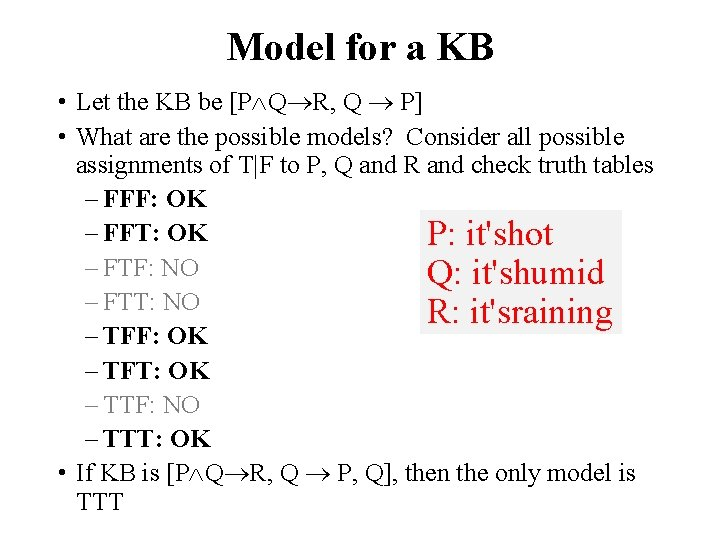Model for a KB • Let the KB be [P Q R, Q P] • What are the possible models? Consider all possible assignments of T|F to P, Q and R and check truth tables – FFF: OK – FFT: OK P: it'shot – FTF: NO Q: it'shumid – FTT: NO R: it'sraining – TFF: OK – TFT: OK – TTF: NO – TTT: OK • If KB is [P Q R, Q P, Q], then the only model is TTT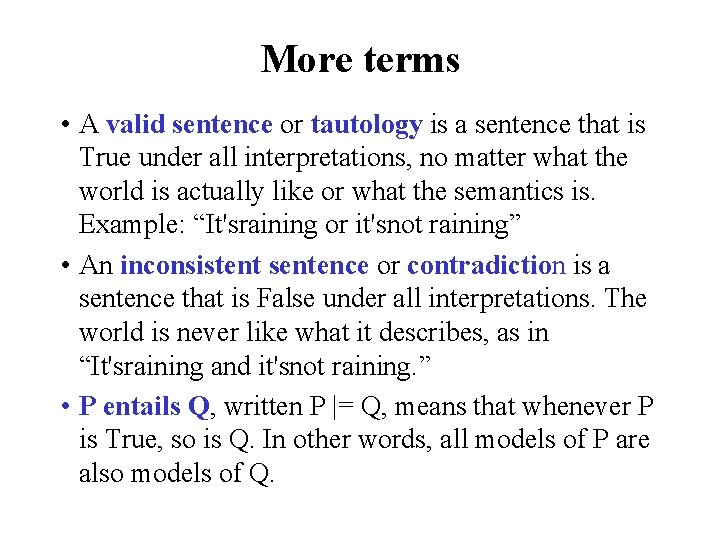More terms • A valid sentence or tautology is a sentence that is True under all interpretations, no matter what the world is actually like or what the semantics is. Example: “It'sraining or it'snot raining” • An inconsistent sentence or contradiction is a sentence that is False under all interpretations. The world is never like what it describes, as in “It'sraining and it'snot raining. ” • P entails Q, written P |= Q, means that whenever P is True, so is Q. In other words, all models of P are also models of Q.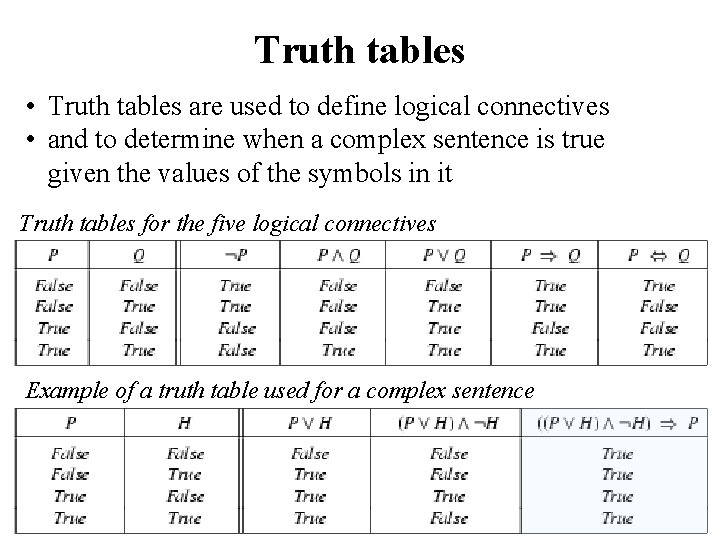Truth tables • Truth tables are used to define logical connectives • and to determine when a complex sentence is true given the values of the symbols in it Truth tables for the five logical connectives Example of a truth table used for a complex sentence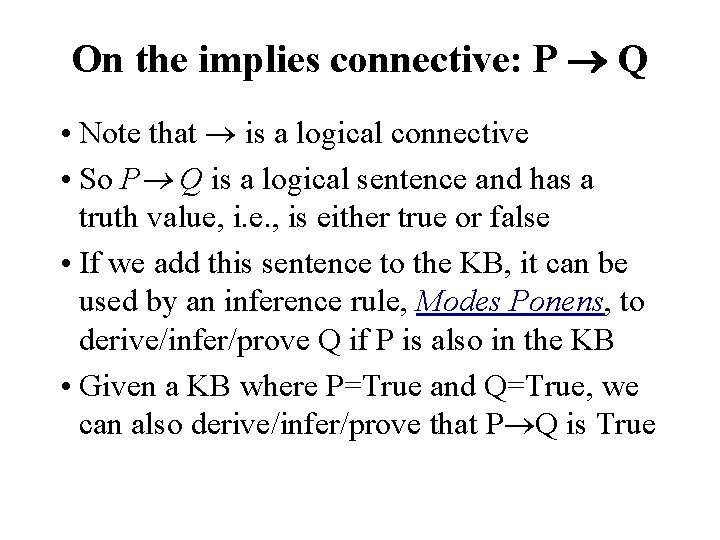On the implies connective: P Q • Note that is a logical connective • So P Q is a logical sentence and has a truth value, i. e. , is either true or false • If we add this sentence to the KB, it can be used by an inference rule, Modes Ponens, to derive/infer/prove Q if P is also in the KB • Given a KB where P=True and Q=True, we can also derive/infer/prove that P Q is True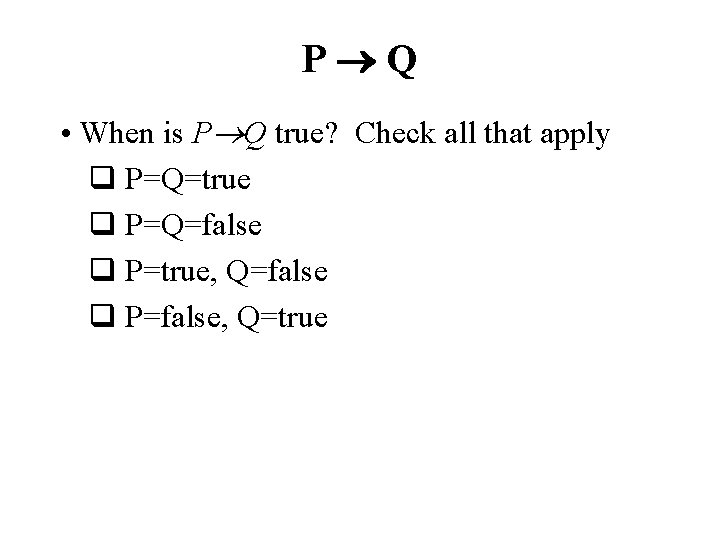P Q • When is P Q true? Check all that apply q P=Q=true q P=Q=false q P=true, Q=false q P=false, Q=trueP Q • When is P Q true? Check all that apply ✔ P=Q=true q ✔ P=Q=false q q P=true, Q=false ✔ P=false, Q=true q • We can get this from the truth table for • Note: in FOL it'smuch harder to prove that a conditional true. – Consider proving prime(x) odd(x)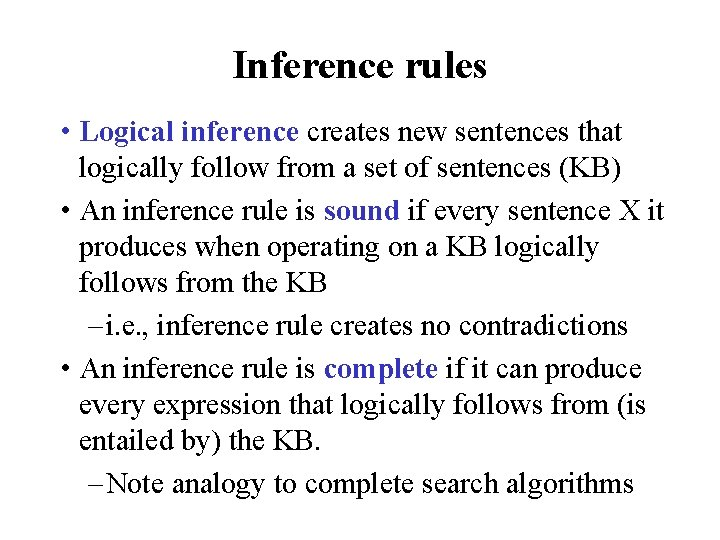Inference rules • Logical inference creates new sentences that logically follow from a set of sentences (KB) • An inference rule is sound if every sentence X it produces when operating on a KB logically follows from the KB – i. e. , inference rule creates no contradictions • An inference rule is complete if it can produce every expression that logically follows from (is entailed by) the KB. – Note analogy to complete search algorithmsSound rules of inference • Here are some examples of sound rules of inference • Each can be shown to be sound using a truth table RULE PREMISE CONCLUSION Modus Ponens And Introduction And Elimination Double Negation Unit Resolution A, A B A, B A B A A B, B C B A A A A C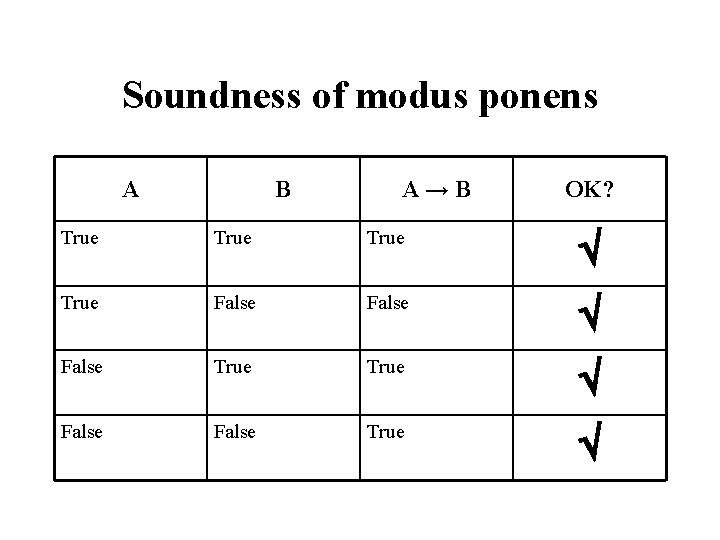Soundness of modus ponens A B A→B OK? True False True False True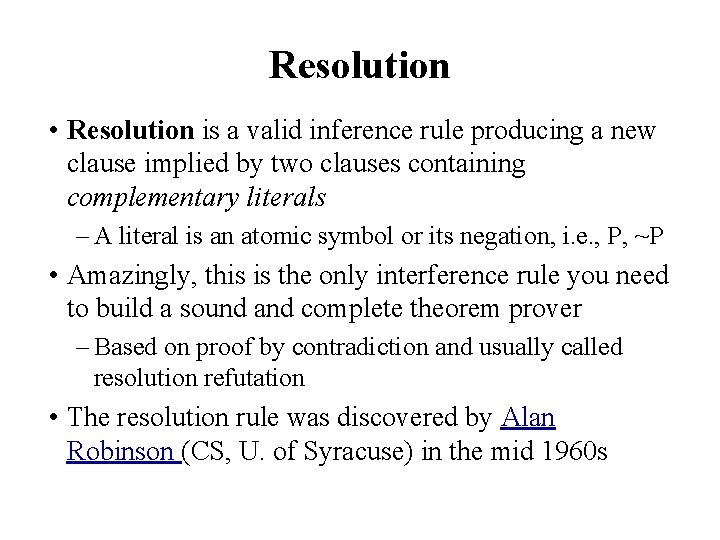Resolution • Resolution is a valid inference rule producing a new clause implied by two clauses containing complementary literals – A literal is an atomic symbol or its negation, i. e. , P, ~P • Amazingly, this is the only interference rule you need to build a sound and complete theorem prover – Based on proof by contradiction and usually called resolution refutation • The resolution rule was discovered by Alan Robinson (CS, U. of Syracuse) in the mid 1960 sResolution • A KB is actually a set of sentences all of which are true, i. e. , a conjunction of sentences. • To use resolution, put KB into conjunctive nor-mal form (CNF), where each sentence written as a disjunction of (one or more) literals • Every KB can be put into CNF, it's just a matter of rewriting its sentences using standard tau-tological rules – (A B)↔(~A B) – (A (B C)) ↔(A B) (A C) – A B A – A B B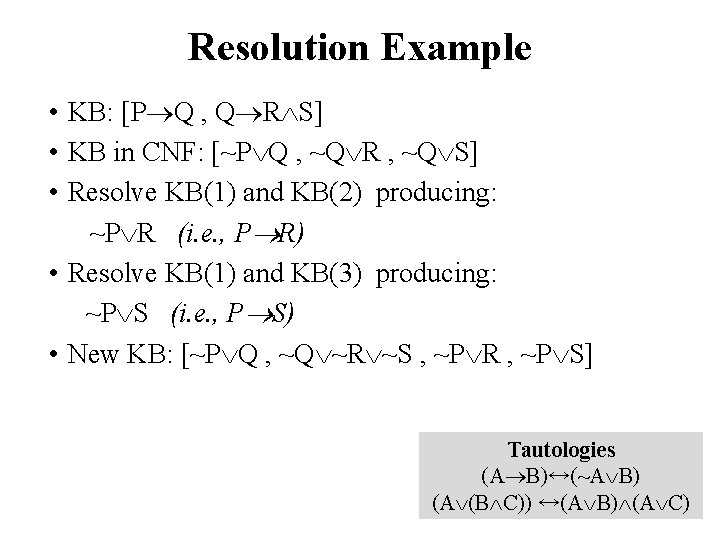Resolution Example • KB: [P Q , Q R S] • KB in CNF: [~P Q , ~Q R , ~Q S] • Resolve KB(1) and KB(2) producing: ~P R (i. e. , P R) • Resolve KB(1) and KB(3) producing: ~P S (i. e. , P S) • New KB: [~P Q , ~Q ~R ~S , ~P R , ~P S] Tautologies (A B)↔(~A B) (A (B C)) ↔(A B) (A C)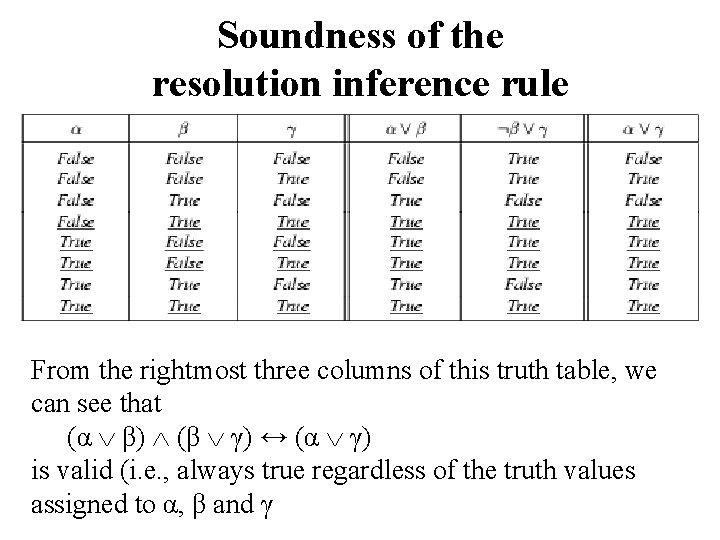Soundness of the resolution inference rule From the rightmost three columns of this truth table, we can see that (α β) (β γ) ↔ (α γ) is valid (i. e. , always true regardless of the truth values assigned to α, β and γ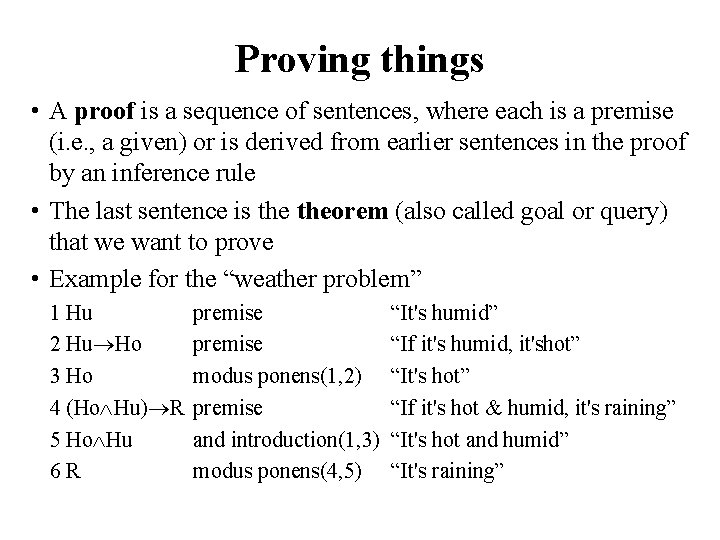Proving things • A proof is a sequence of sentences, where each is a premise (i. e. , a given) or is derived from earlier sentences in the proof by an inference rule • The last sentence is theorem (also called goal or query) that we want to prove • Example for the “weather problem” 1 Hu 2 Hu Ho 3 Ho 4 (Ho Hu) R 5 Ho Hu 6 R premise modus ponens(1, 2) premise and introduction(1, 3) modus ponens(4, 5) “It's humid” “If it's humid, it'shot” “It's hot” “If it's hot & humid, it's raining” “It's hot and humid” “It's raining”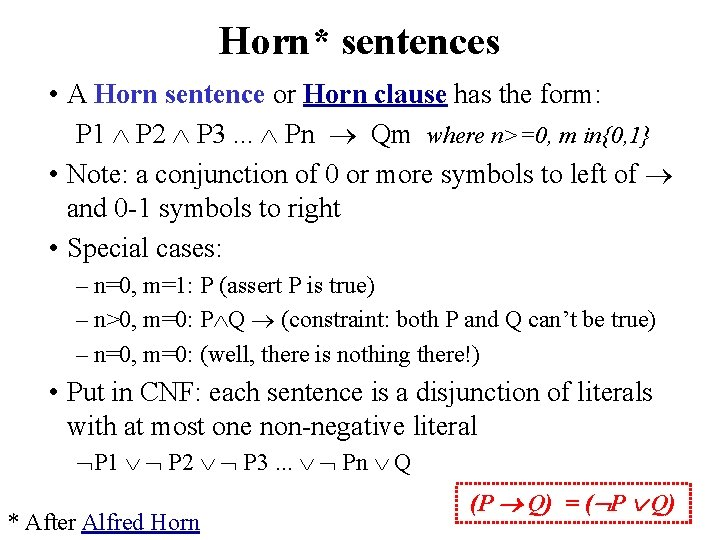Horn* sentences • A Horn sentence or Horn clause has the form: P 1 P 2 P 3. . . Pn Qm where n>=0, m in{0, 1} • Note: a conjunction of 0 or more symbols to left of and 0 -1 symbols to right • Special cases: – n=0, m=1: P (assert P is true) – n>0, m=0: P Q (constraint: both P and Q can’t be true) – n=0, m=0: (well, there is nothing there!) • Put in CNF: each sentence is a disjunction of literals with at most one non-negative literal P 1 P 2 P 3. . . Pn Q * After Alfred Horn (P Q) = ( P Q)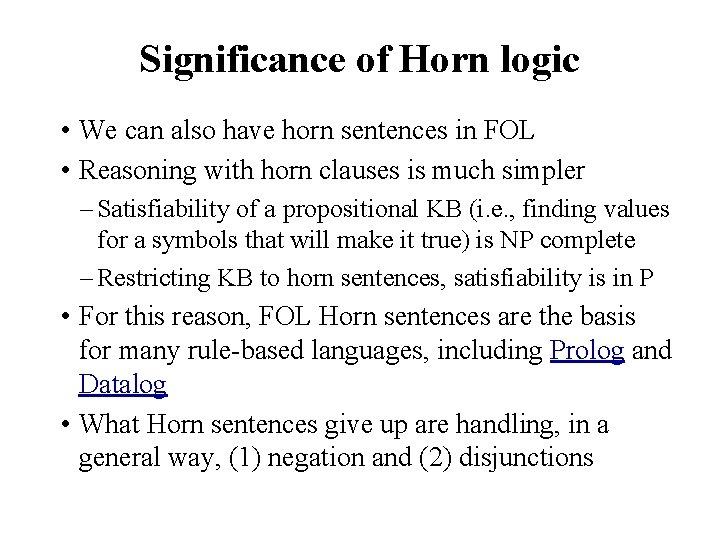Significance of Horn logic • We can also have horn sentences in FOL • Reasoning with horn clauses is much simpler – Satisfiability of a propositional KB (i. e. , finding values for a symbols that will make it true) is NP complete – Restricting KB to horn sentences, satisfiability is in P • For this reason, FOL Horn sentences are the basis for many rule-based languages, including Prolog and Datalog • What Horn sentences give up are handling, in a general way, (1) negation and (2) disjunctionsEntailment and derivation • Entailment: KB |= Q – Q is entailed by KB (set sentences) iff there is no logically possible world where Q is false while all the sentences in KB are true – Or, stated positively, Q is entailed by KB iff the conclusion is true in every logically possible world in which all the premises in KB are true • Derivation: KB |- Q – We can derive Q from KB if there's a proof consisting of a sequence of valid inference steps starting from the premises in KB and resulting in QTwo important properties for inference Soundness: If KB |- Q then KB |= Q – If Q is derived from KB using a given set of rules of inference, then Q is entailed by KB – Hence, inference produces only real entailments, or any sentence that follows deductively from the premises is valid Completeness: If KB |= Q then KB |- Q – If Q is entailed by KB, then Q can be derived from KB using the rules of inference – Hence, inference produces all entailments, or all valid sentences can be proved from the premisesProblems with Propositional Logic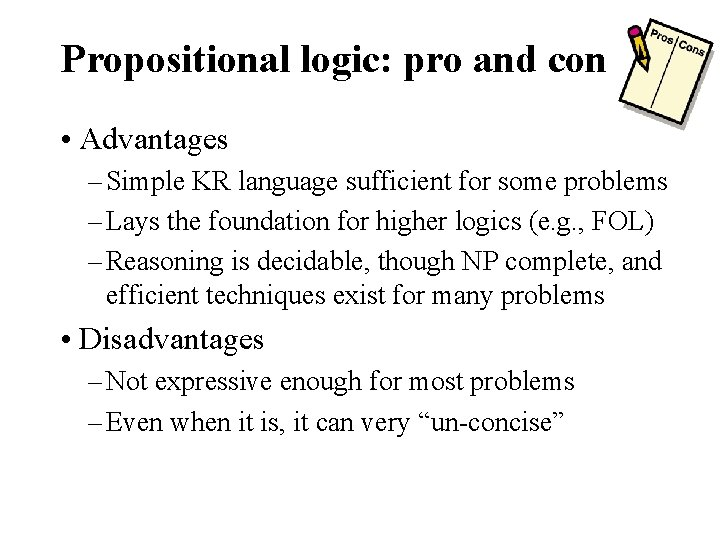Propositional logic: pro and con • Advantages – Simple KR language sufficient for some problems – Lays the foundation for higher logics (e. g. , FOL) – Reasoning is decidable, though NP complete, and efficient techniques exist for many problems • Disadvantages – Not expressive enough for most problems – Even when it is, it can very “un-concise”PL is a weak KR language • Hard to identify “individuals” (e. g. , Mary, 3) • Can’t directly talk about properties of individuals or relations between individuals (e. g. , “Bill is tall”) • Generalizations, patterns, regularities can’t easily be represented (e. g. , “all triangles have 3 sides”) • First-Order Logic (FOL) is expressive enough to represent this kind of information using relations, variables and quantifiers, e. g. , • Every elephant is gray: x (elephant(x) → gray(x)) • There is a white alligator: x (alligator(X) ^ white(X))Hunt the Wumpus domain • Some atomic propositions: S 12 = There is a stench in cell (1, 2) B 34 = There is a breeze in cell (3, 4) W 22 = Wumpus is in cell (2, 2) V 11 = We’ve visited cell (1, 1) OK 11 = Cell (1, 1) is safe … • Some rules: S 22 W 12 W 23 W 32 W 21 B 22 P 12 P 23 P 32 P 21 W 22 S 12 S 23 W 21 W 22 W 11 W 21 … W 44 A 22 V 22 A 22 W 11 W 21 … W 44 V 22 OK 22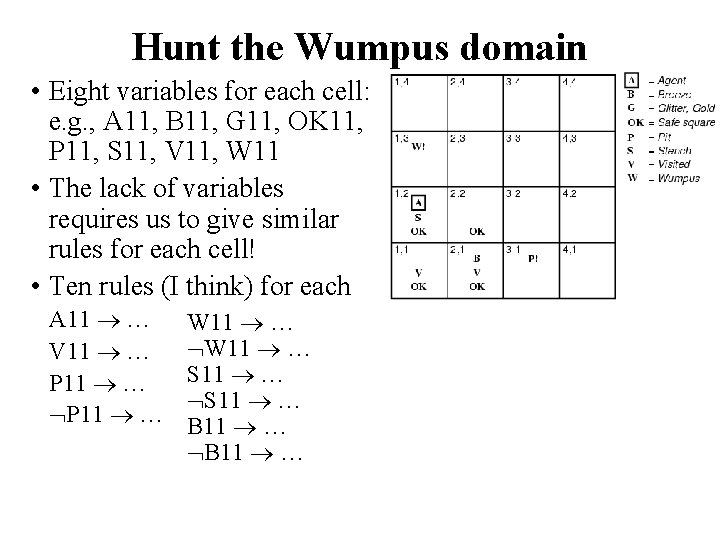Hunt the Wumpus domain • Eight variables for each cell: e. g. , A 11, B 11, G 11, OK 11, P 11, S 11, V 11, W 11 • The lack of variables requires us to give similar rules for each cell! • Ten rules (I think) for each A 11 … V 11 … P 11 … W 11 … S 11 … B 11 …After third move • We can prove that the Wumpus is in (1, 3) using these four rules • See R&N section 7. 5 (R 1) S 11 W 11 W 12 W 21 (R 2) S 21 W 11 W 22 W 31 (R 3) S 12 W 11 W 12 W 22 W 13 (R 4) S 12 W 13 W 12 W 22 W 11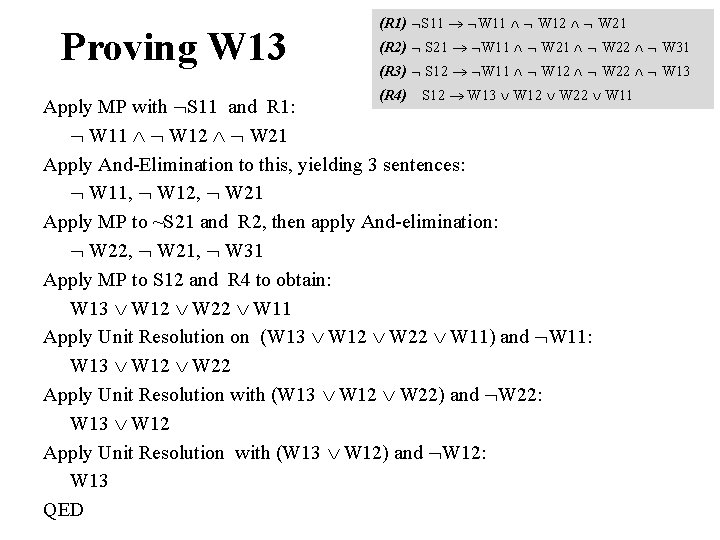Proving W 13 (R 1) S 11 W 11 W 12 W 21 (R 2) S 21 W 11 W 22 W 31 (R 3) S 12 W 11 W 12 W 22 W 13 (R 4) S 12 W 13 W 12 W 22 W 11 Apply MP with S 11 and R 1: W 11 W 12 W 21 Apply And-Elimination to this, yielding 3 sentences: W 11, W 12, W 21 Apply MP to ~S 21 and R 2, then apply And-elimination: W 22, W 21, W 31 Apply MP to S 12 and R 4 to obtain: W 13 W 12 W 22 W 11 Apply Unit Resolution on (W 13 W 12 W 22 W 11) and W 11: W 13 W 12 W 22 Apply Unit Resolution with (W 13 W 12 W 22) and W 22: W 13 W 12 Apply Unit Resolution with (W 13 W 12) and W 12: W 13 QED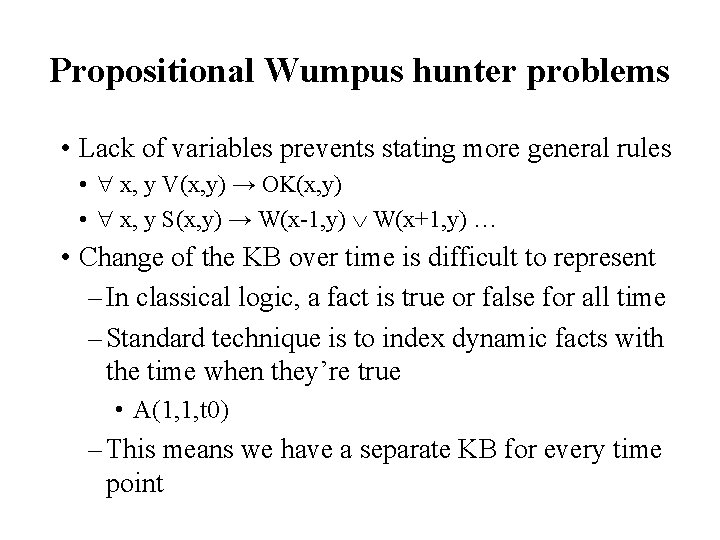Propositional Wumpus hunter problems • Lack of variables prevents stating more general rules • x, y V(x, y) → OK(x, y) • x, y S(x, y) → W(x-1, y) W(x+1, y) … • Change of the KB over time is difficult to represent – In classical logic, a fact is true or false for all time – Standard technique is to index dynamic facts with the time when they’re true • A(1, 1, t 0) – This means we have a separate KB for every time point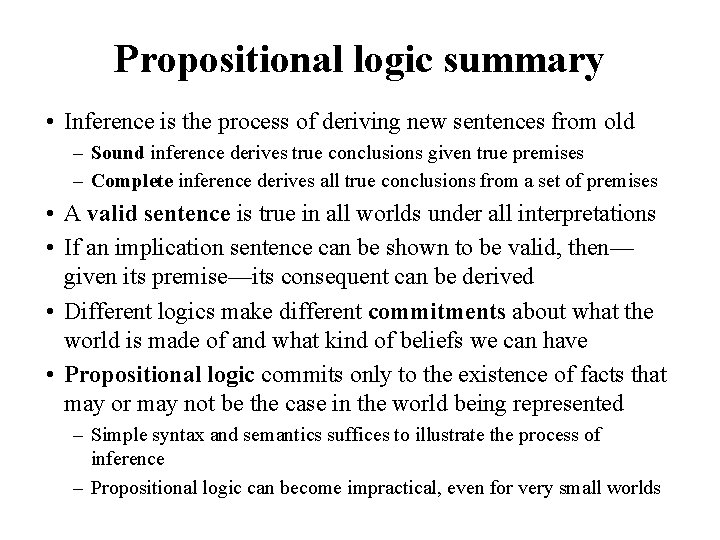Propositional logic summary • Inference is the process of deriving new sentences from old – Sound inference derives true conclusions given true premises – Complete inference derives all true conclusions from a set of premises • A valid sentence is true in all worlds under all interpretations • If an implication sentence can be shown to be valid, then— given its premise—its consequent can be derived • Different logics make different commitments about what the world is made of and what kind of beliefs we can have • Propositional logic commits only to the existence of facts that may or may not be the case in the world being represented – Simple syntax and semantics suffices to illustrate the process of inference – Propositional logic can become impractical, even for very small worlds